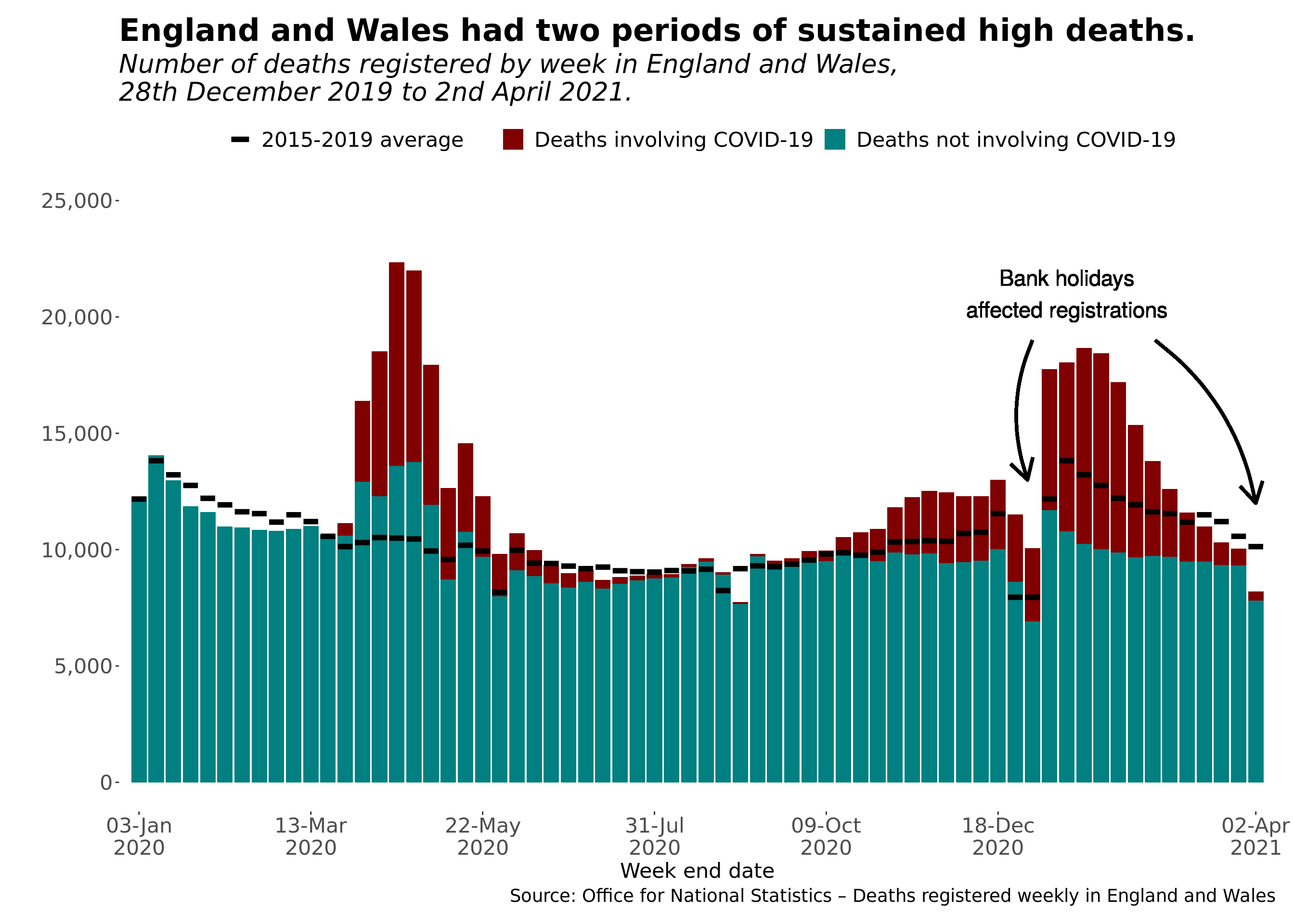# Graphing mortality II

## On bar graphs, showing a value using a line can be effective.

Last week, I looked at how to emulate the mortality graph with a ranged ribbon. This week, I seek to emulate a graph in the Office for National Statistics weekly death reports.

The graph has the following key elements:

• A stacked bar graph, showing deaths which involve and do not involve COVID-19. A death ‘involves’ a disease if clinicians believe it caused or contributed to the death.
• A straight line representing the weekly average of deaths in 2015 to 2019.
• A legend showing what all three counts correspond to on the graph.
• Informative text and arrows, highlighting public holidays influence death registrations in particular weeks.

# Setting up

First, we start by install packages that we need:

`library(tidyverse)library(readxl)library(scales)library(lubridate)`

I had some trouble installing the ‘ungeviz’ package in R Studio Cloud. I was able to find Prof Wilke’s code for the `geom_hpline` function. We can use that instead. We draw the values from a prepared file (which I added a date to):

`ons_deathregistration_figure3_df <- read_excel("ONS Weekly Death Registrations Figure 3 - 2021-04-14.xlsx",                                              sheet = "DATA",                                              col_types = c("numeric", "text", "date", "numeric", "numeric", "numeric"))`

Next, we tidy that data set. Each of the three measures is its own row for each week:

`ons_deathreg_tidy_df <- ons_deathregistration_figure3_df %>%  mutate(week_end_date = as_date(week_end_date)) %>%  pivot_longer(cols = 4:6,               names_to = "ons_measure",               values_to = "count")`

# Creating the graph

We set the date breaks to appear on the graph:

`ons_week_breaks <- c("2020-01-03", "2020-03-13", "2020-05-22", "2020-07-31", "2020-10-09", "2020-12-18", "2021-04-02") %>%  as_date()`

The code for the graph is then made up of several components. This is the core for the stacked bar graph:

`ons_deathreg_figure3_gg <-  ggplot(data = filter(ons_deathreg_tidy_df,                       ons_measure != "all_deaths_2015_2019_average"),         aes(x = week_end_date)) +  geom_bar(aes(y = count,               fill = ons_measure),           position = "stack",           stat = "identity") +`

Next, we add the line representing the past weekly average. Even though there is only one value, we want the legend for this measure. That is the purpose of setting the line-type:

`geom_hpline(data = filter(ons_deathreg_tidy_df,                            ons_measure == "all_deaths_2015_2019_average"),              aes(x = week_end_date,                  y = count,                  linetype = ons_measure),              stat = "identity",              width = 6, size = 2) +`

On each axis, we make the scales look pretty. Dates have familiar format, including the year on the new line:

`scale_x_date(breaks = ons_week_breaks,               date_labels = "%d-%b\n%Y",               expand = c(0,5)) +  scale_y_continuous(labels = label_comma(),                     limits = c(0,25000)) +`

The following lines determine the colours and what we see in the legend:

`scale_linetype_manual(name = "",                        labels = "2015-2019 average",                        values = "solid") +  scale_fill_manual(name = "",                    labels = c("Deaths involving COVID-19", "Deaths not involving COVID-19"),                    values = c("#800000", "#008080")) +`

Almost there. Next, we add the title labels, including removing a label for the vertical axis. The `str_wrap` function contains the subtitle.

`labs(title = "England and Wales had two periods of sustained high deaths.",       subtitle = str_wrap("Number of deaths registered by week in England and Wales, 28th December 2019 to 2nd April 2021.", width = 60),       x = "Week end date",       y = "",       caption = "Source: Office for National Statistics – Deaths registered weekly in England and Wales") +`

Finally, we want to add some text and arrows. This takes some trial-and-error to get right:

`geom_text(x = as_date("2021-01-15"), y = 21000,            label = "Bank holidays\naffected registrations",            size = 6) +  geom_curve(x = as_date("2021-01-01"), xend = as_date("2020-12-30"),             y = 19000, yend = 13000,             arrow = arrow(), curvature = 0.2, size = 1.2) +  geom_curve(x = as_date("2021-02-20"), xend = as_date("2021-04-02"),             y = 19000, yend = 12000,             arrow = arrow(), curvature = -0.2, size = 1.2)`

The result of all that code is this graph:That is like the original graph. Adjustments to the theme and line type would bring us closer. (Image: R Pubs)

The full R code is available on R Pubs and GitHub.

This blog looks at the use of statistics in Britain and beyond. It is written by RSS Statistical Ambassador and Chartered Statistician @anthonybmasters.

## More from Anthony B. Masters

This blog looks at the use of statistics in Britain and beyond. It is written by RSS Statistical Ambassador and Chartered Statistician @anthonybmasters.